# Recursion Chapter 11 Recursion Review of Methods n

• Slides: 68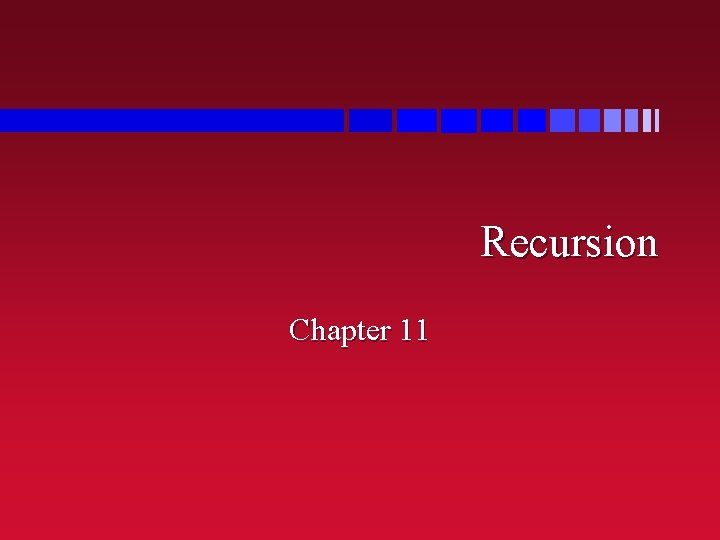Recursion Chapter 11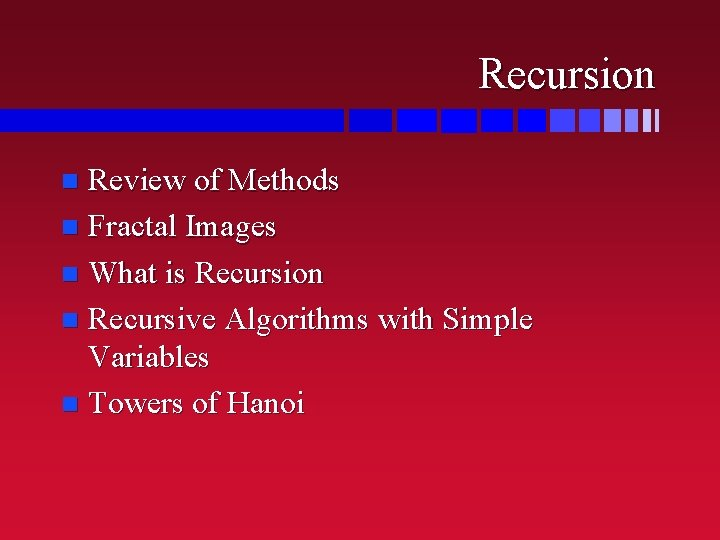Recursion Review of Methods n Fractal Images n What is Recursion n Recursive Algorithms with Simple Variables n Towers of Hanoi n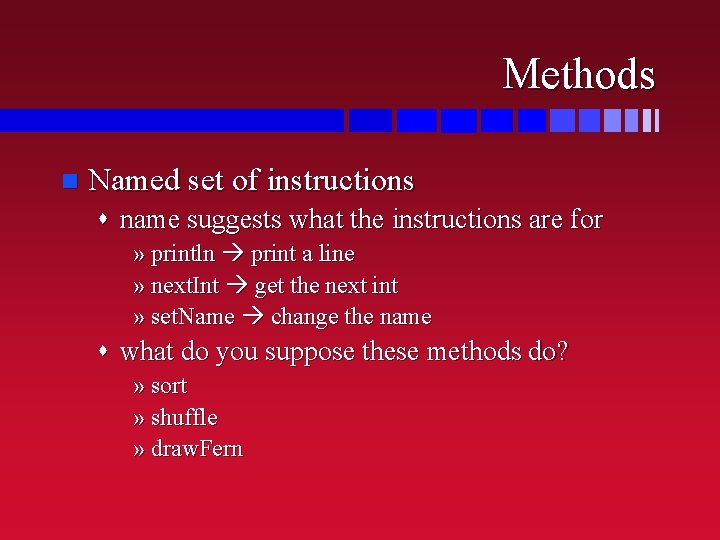Methods n Named set of instructions s name suggests what the instructions are for » println print a line » next. Int get the next int » set. Name change the name s what do you suppose these methods do? » sort » shuffle » draw. FernThe Job of the Method n Every method has a job to do s print a line, get the next int, draw a fern, … » (part of the job may be signalling errors) n Call the method the job gets done s that’s what the method is there for n How the method does the job… s the body/definition of the method n …is none of the caller’s business!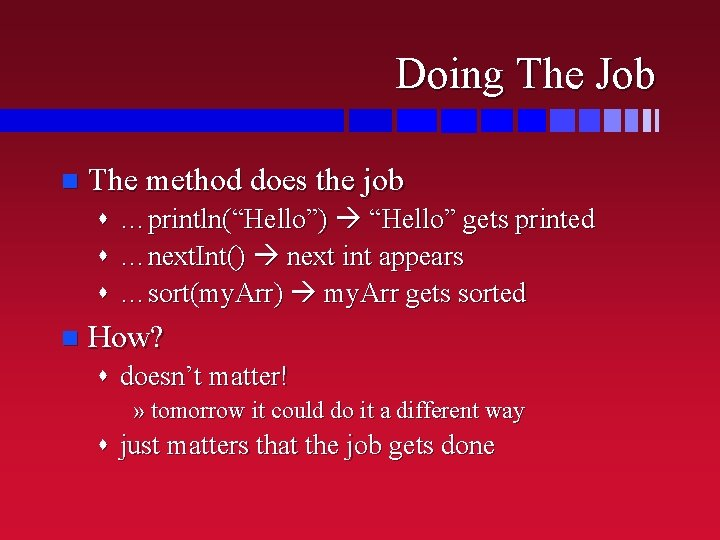Doing The Job n The method does the job s …println(“Hello”) “Hello” gets printed s …next. Int() next int appears s …sort(my. Arr) my. Arr gets sorted n How? s doesn’t matter! » tomorrow it could do it a different way s just matters that the job gets doneArguments and Parameters n Methods often need extra information s what do you want me to print on the line? s what do you want me to change the name to? s which array do you want to sort/shuffle? n Extra information given as an argument buddy. set. Name(“Buford”); n Extra information received as a parameter public void set. Name(String new. Name) { …}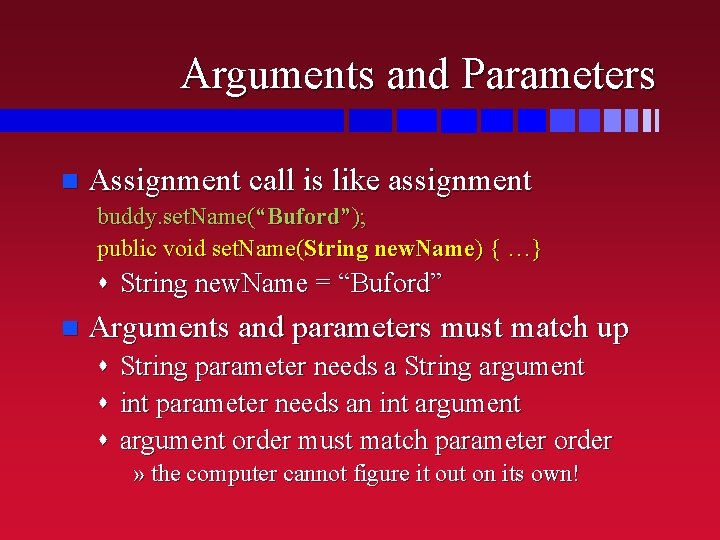Arguments and Parameters n Assignment call is like assignment buddy. set. Name(“Buford”); public void set. Name(String new. Name) { …} s String new. Name = “Buford” n Arguments and parameters must match up s String parameter needs a String argument s int parameter needs an int argument s argument order must match parameter order » the computer cannot figure it out on its own!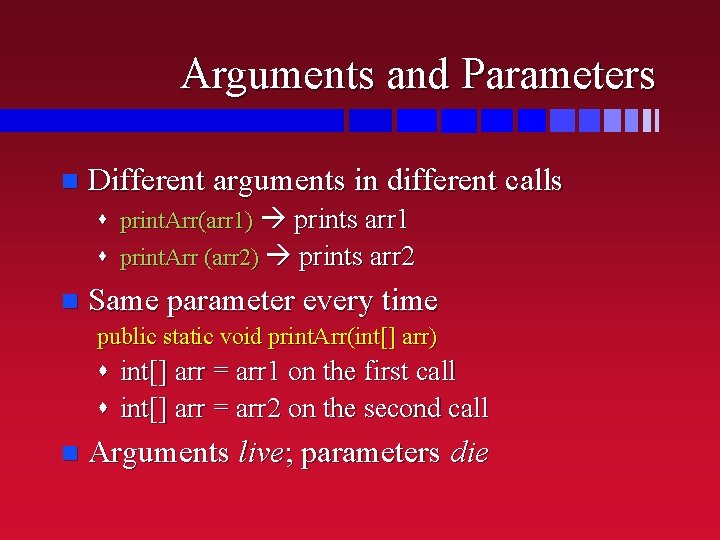Arguments and Parameters n Different arguments in different calls s print. Arr(arr 1) prints arr 1 s print. Arr (arr 2) prints arr 2 n Same parameter every time public static void print. Arr(int[] arr) s int[] arr = arr 1 on the first call s int[] arr = arr 2 on the second call n Arguments live; parameters dieMethods and Arguments The method does its job… n …with the arguments you gave it… n s it sorts the array you told it to sort s it prints the line you told it to print s it draws the fern in the place you told it to draw the fern n …because that’s its job! REMEMBER THAT!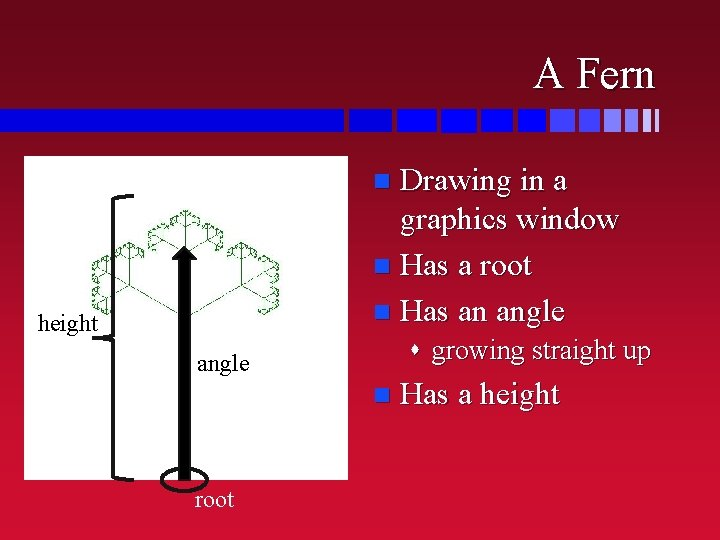A Fern Drawing in a graphics window n Has a root n Has an angle n height s growing straight up angle n root Has a heightThe draw. Fern Method n A method to draw a fern draw. Fern(double x, double y, double angle, double size) s say where we want the bottom of the fern to be » how many pixels across (x) and down (y) s say what angle we want the fern drawn at » straight up = 180º, flat out to right = 90º s say how big we want the fern to be » how many pixels from base to tip n This method draws a fern – that’s its job!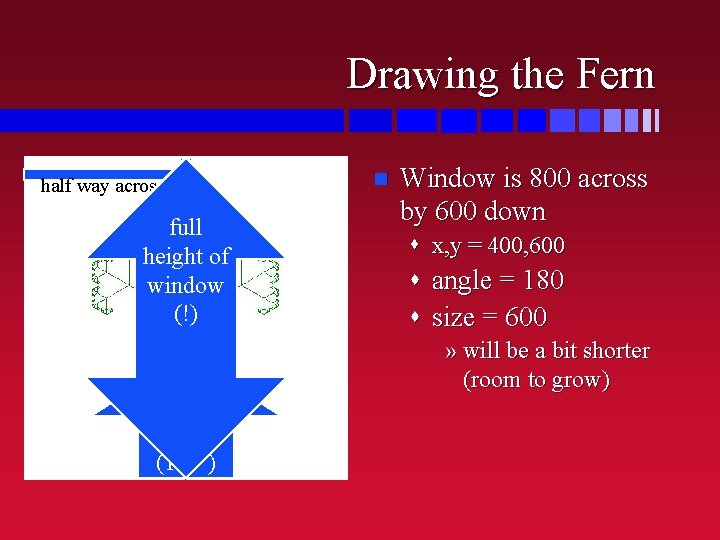Drawing the Fern half way across all the way down full height of window (!) straight up (180º) n Window is 800 across by 600 down s x, y = 400, 600 s angle = 180 s size = 600 » will be a bit shorter (room to grow)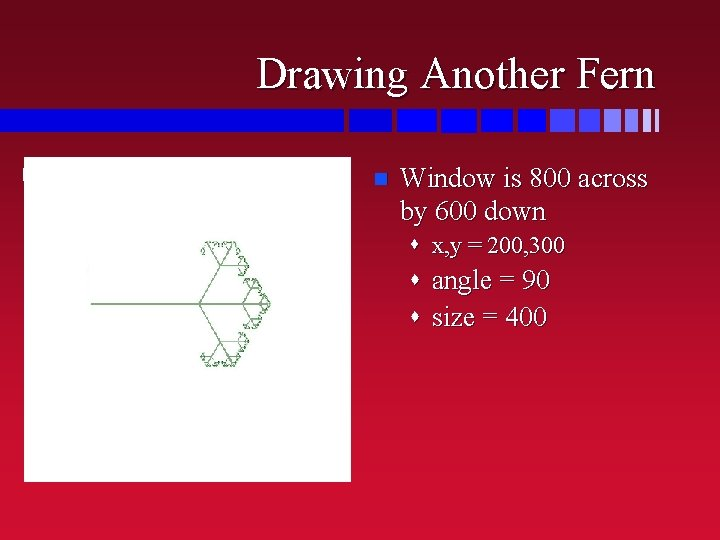Drawing Another Fern n half way down 1/4 way across Window is 800 across by 600 down s x, y = 200, 300 half width off to of side window (90º) s angle = 90 s size = 400Exercise: Draw this Fern n Window is 800 across by 600 down s root is at centre of window s angle is 120 degrees s height is 40% of the window’s height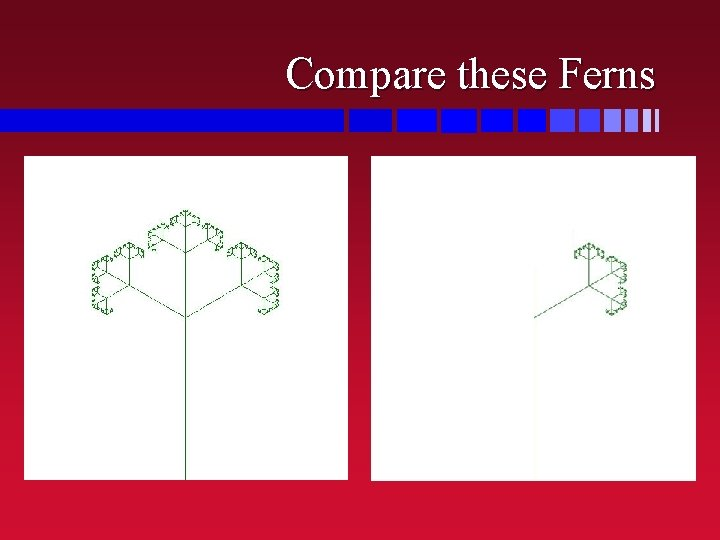Compare these Ferns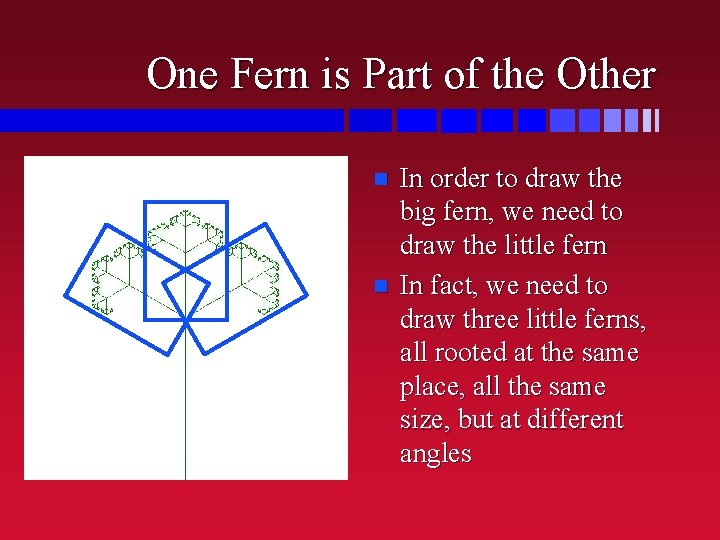One Fern is Part of the Other n n In order to draw the big fern, we need to draw the little fern In fact, we need to draw three little ferns, all rooted at the same place, all the same size, but at different angles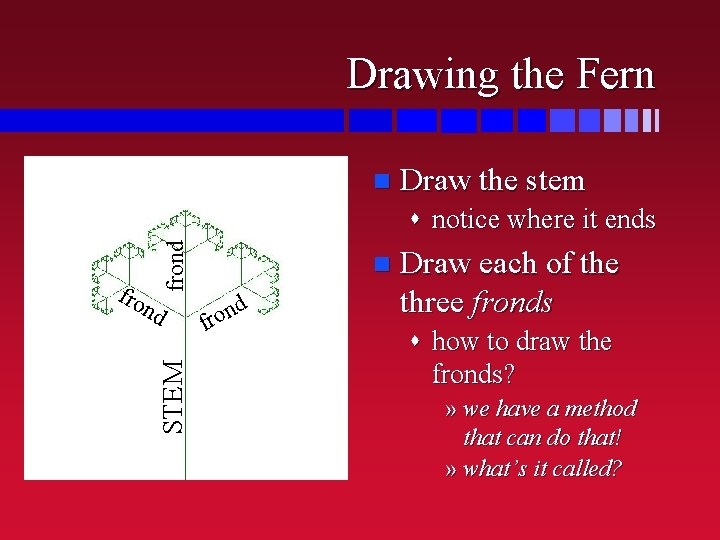Drawing the Fern n Draw the stem fro n frond s notice where it ends STEM d n o fr Draw each of the three fronds s how to draw the fronds? » we have a method that can do that! » what’s it called?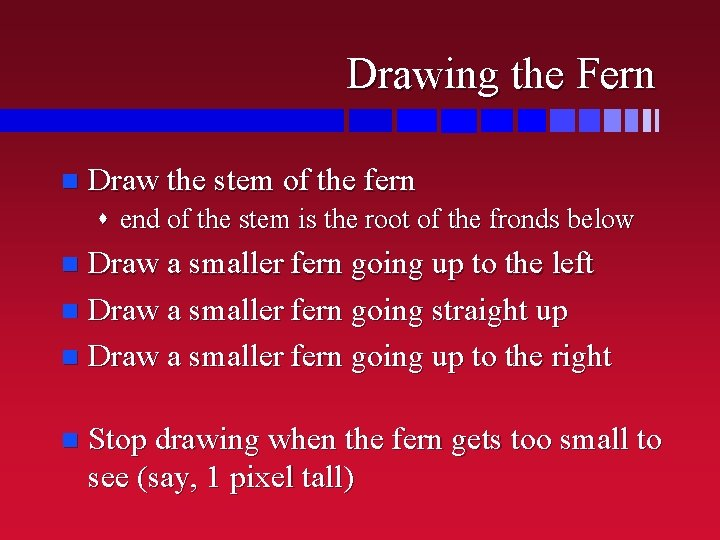Drawing the Fern n Draw the stem of the fern s end of the stem is the root of the fronds below Draw a smaller fern going up to the left n Draw a smaller fern going straight up n Draw a smaller fern going up to the right n n Stop drawing when the fern gets too small to see (say, 1 pixel tall)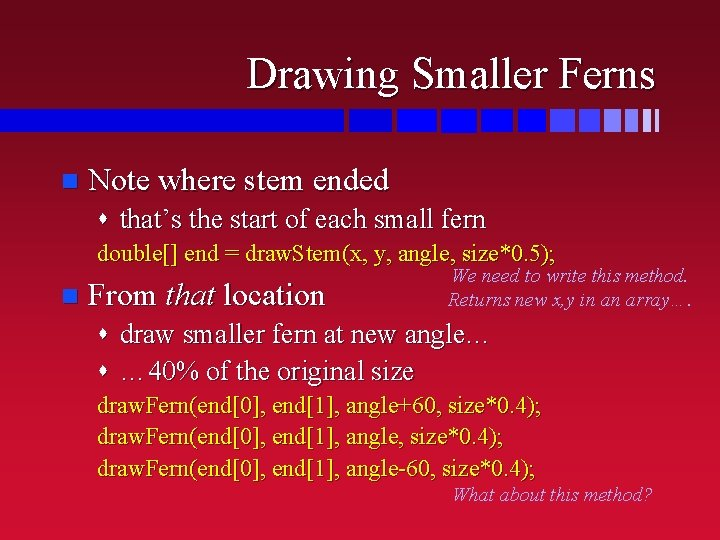Drawing Smaller Ferns n Note where stem ended s that’s the start of each small fern double[] end = draw. Stem(x, y, angle, size*0. 5); n From that location We need to write this method. Returns new x, y in an array…. s draw smaller fern at new angle… s … 40% of the original size draw. Fern(end, end, angle+60, size*0. 4); draw. Fern(end, end, angle-60, size*0. 4); What about this method?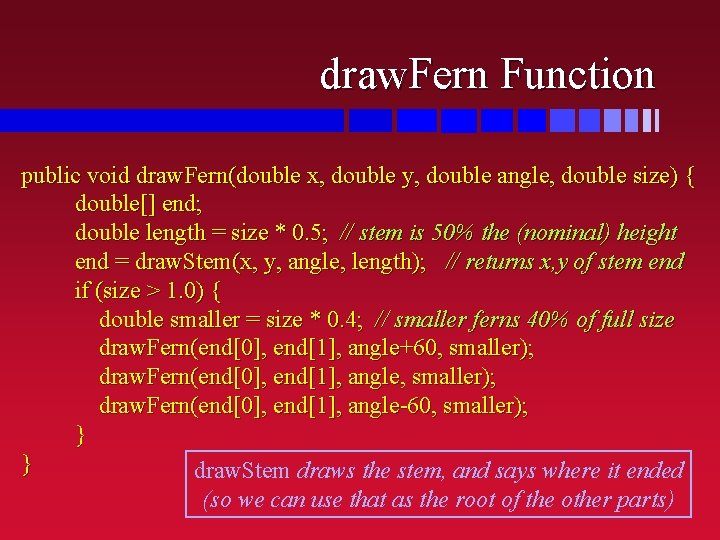draw. Fern Function public void draw. Fern(double x, double y, double angle, double size) { double[] end; double length = size * 0. 5; // stem is 50% the (nominal) height end = draw. Stem(x, y, angle, length); // returns x, y of stem end if (size > 1. 0) { double smaller = size * 0. 4; // smaller ferns 40% of full size draw. Fern(end, end, angle+60, smaller); draw. Fern(end, end, angle-60, smaller); } } draw. Stem draws the stem, and says where it ended (so we can use that as the root of the other parts)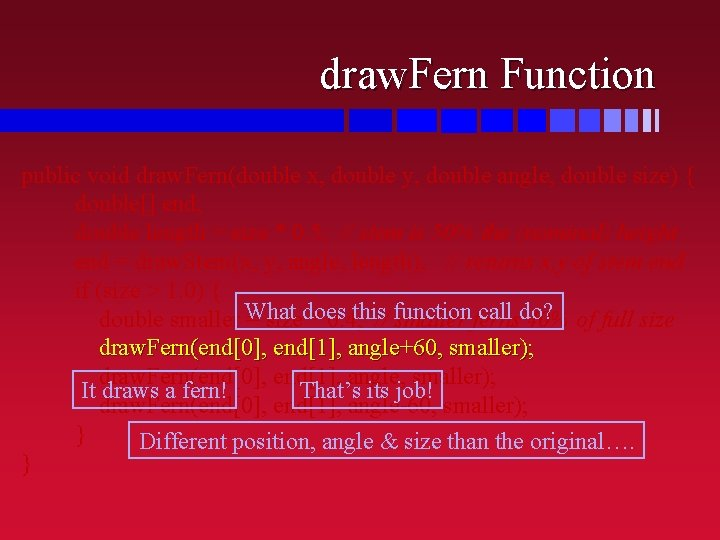draw. Fern Function public void draw. Fern(double x, double y, double angle, double size) { double[] end; double length = size * 0. 5; // stem is 50% the (nominal) height end = draw. Stem(x, y, angle, length); // returns x, y of stem end if (size > 1. 0) { call do? double smaller What = sizedoes * 0. 4; this// function smaller ferns 40% of full size draw. Fern(end, end, angle+60, smaller); draw. Fern(end, end, angle, smaller); It draws a fern! That’s its job! draw. Fern(end, end, angle-60, smaller); } Different position, angle & size than the original…. }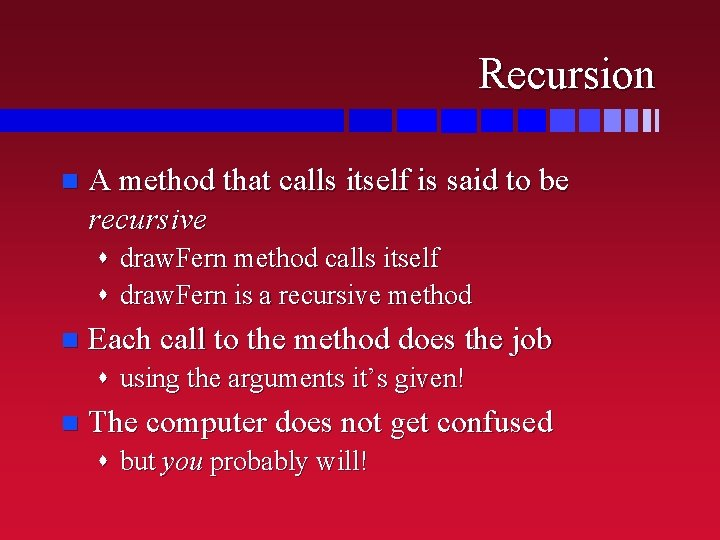Recursion n A method that calls itself is said to be recursive s draw. Fern method calls itself s draw. Fern is a recursive method n Each call to the method does the job s using the arguments it’s given! n The computer does not get confused s but you probably will!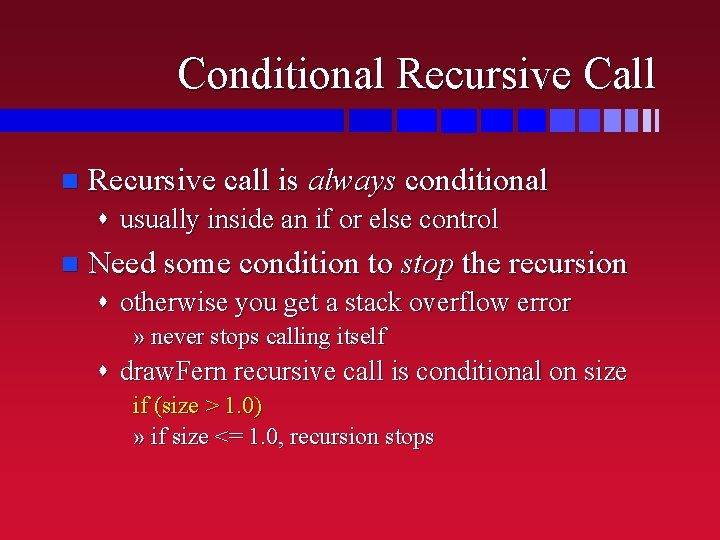Conditional Recursive Call n Recursive call is always conditional s usually inside an if or else control n Need some condition to stop the recursion s otherwise you get a stack overflow error » never stops calling itself s draw. Fern recursive call is conditional on size if (size > 1. 0) » if size <= 1. 0, recursion stops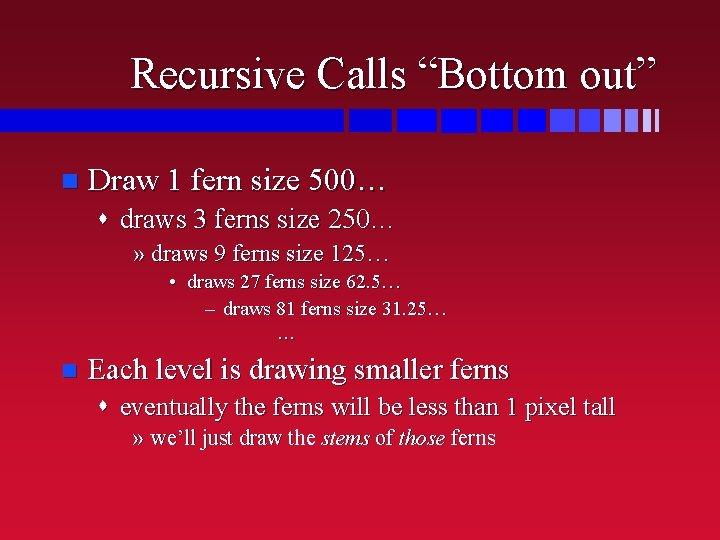Recursive Calls “Bottom out” n Draw 1 fern size 500… s draws 3 ferns size 250… » draws 9 ferns size 125… • draws 27 ferns size 62. 5… – draws 81 ferns size 31. 25… … n Each level is drawing smaller ferns s eventually the ferns will be less than 1 pixel tall » we’ll just draw the stems of those fernsFractals n See the pretty pictures! s http: //en. wikipedia. org/wiki/Fractal s http: //www. geocities. com/rerewhakaaitu/ s Xao. S viewer » you can download this for yourself n These are “self-similar” objects s smaller pieces of self look like whole thing s like our fern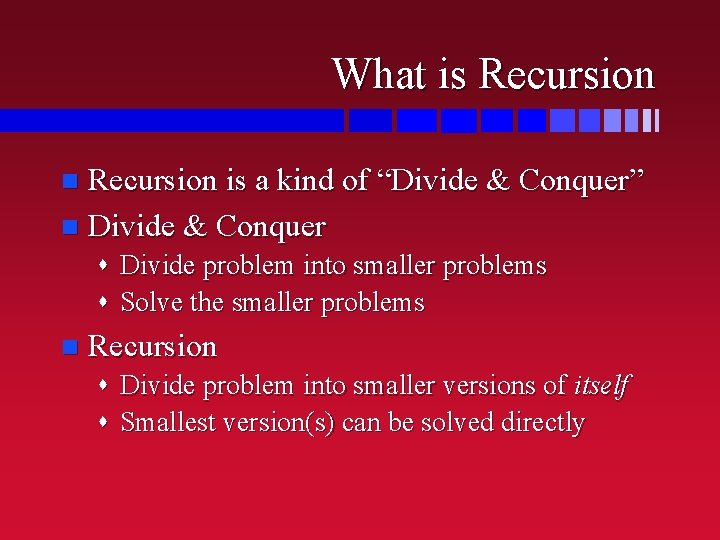What is Recursion is a kind of “Divide & Conquer” n Divide & Conquer n s Divide problem into smaller problems s Solve the smaller problems n Recursion s Divide problem into smaller versions of itself s Smallest version(s) can be solved directly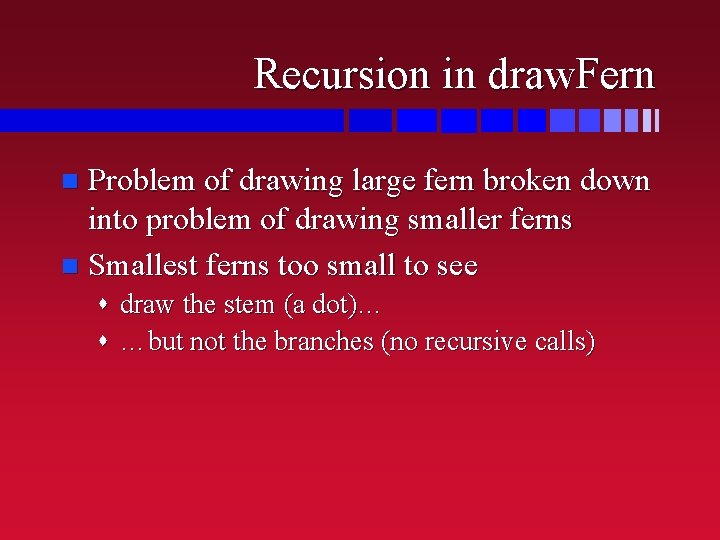Recursion in draw. Fern Problem of drawing large fern broken down into problem of drawing smaller ferns n Smallest ferns too small to see n s draw the stem (a dot)… s …but not the branches (no recursive calls)Koch’s Snowflake Fractal image n Draw a triangle, except… n …on each side n s draw a smaller triangle sticking out n Keep going! s see the animation on wikipedia http: //en. wikipedia. org/wiki/Koch_snowflake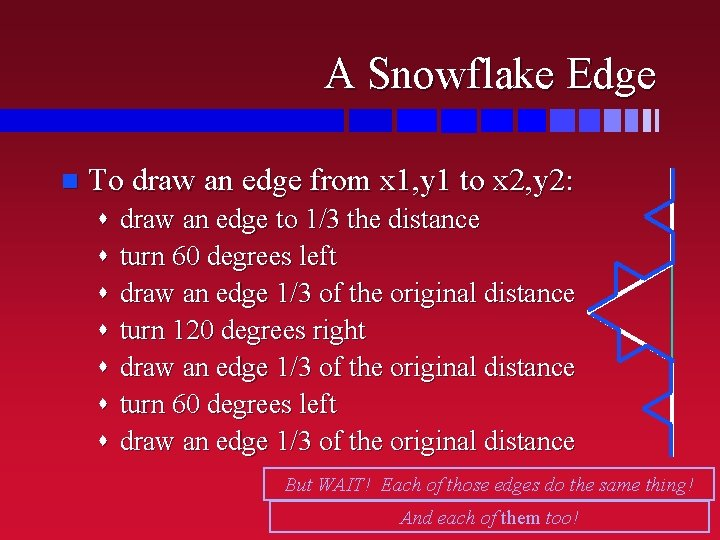A Snowflake Edge n To draw an edge from x 1, y 1 to x 2, y 2: s draw an edge to 1/3 the distance s turn 60 degrees left s draw an edge 1/3 of the original distance s turn 120 degrees right s draw an edge 1/3 of the original distance s turn 60 degrees left s draw an edge 1/3 of the original distance But WAIT! Each of those edges do the same thing! And each of them too!Recursion in the Snowflake n Problem of drawing an edge is broken down into problem of drawing smaller edges s recursion! n Smallest lines just get drawn straight s one or two dots s no recursion s “base case”Drawing the Snowflake Edge n Need to know s how long the basic edge should be s where to start (x, y) s what direction to go in n Return where we stop s so we can start the next edge from there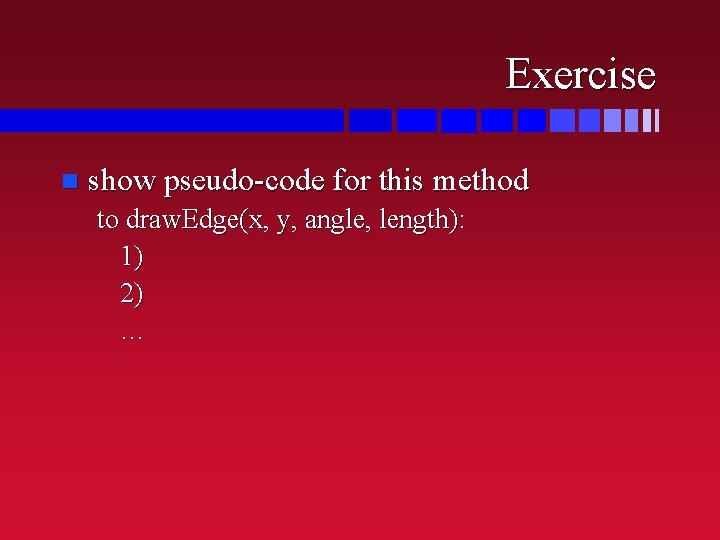Exercise n show pseudo-code for this method to draw. Edge(x, y, angle, length): 1) 2) …Definitions and Recursion Mathematicians like to define things recursively n Recursive definition uses the thing being defined in its own definition! n Step back: conditional definition n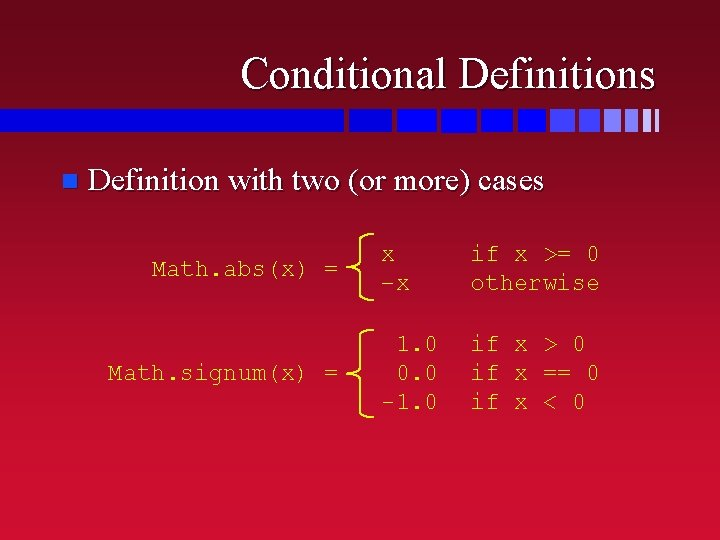Conditional Definitions n Definition with two (or more) cases Math. abs(x) = Math. signum(x) = x –x if x >= 0 otherwise 1. 0 0. 0 -1. 0 if x > 0 if x == 0 if x < 0Recursive Definitions n One (or more) cases use the term being defined n! = Fib(n) = 1 n*(n-1)! if n == 0 if n > 0 0 1 Fib(n– 1)+Fib(n– 2) if n == 0 if n == 1 if n > 1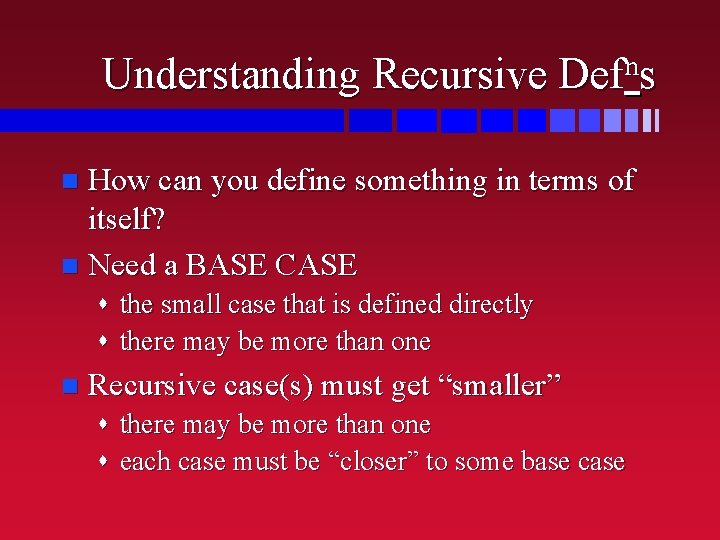Understanding Recursive n Def s How can you define something in terms of itself? n Need a BASE CASE n s the small case that is defined directly s there may be more than one n Recursive case(s) must get “smaller” s there may be more than one s each case must be “closer” to some base caseGetting Smaller 4! = 4 * 3! n 3! = 3 * 2! n 2! = 2 * 1! n 1! = 1 * 0! n 0! = 1 n n! = Recursive Case Base Case 1 n*(n-1)! if n == 0 if n > 0 4 * 6 = 24 3*2=6 2*1=2 1*1=1 Base Case Recursive Case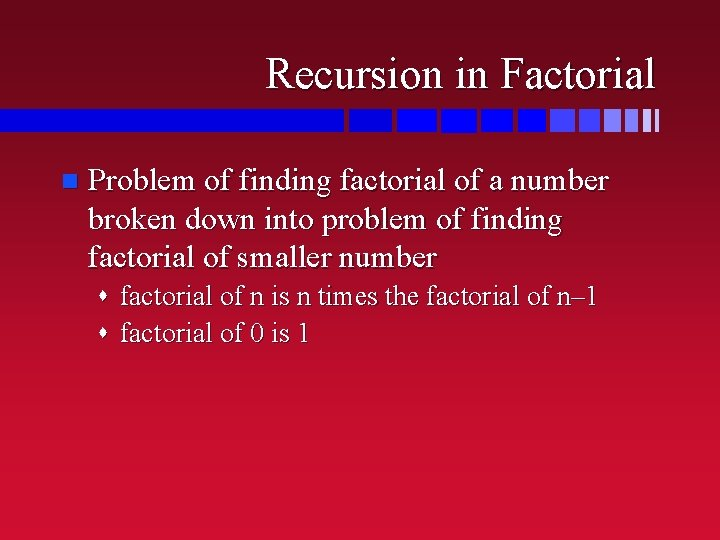Recursion in Factorial n Problem of finding factorial of a number broken down into problem of finding factorial of smaller number s factorial of n is n times the factorial of n– 1 s factorial of 0 is 1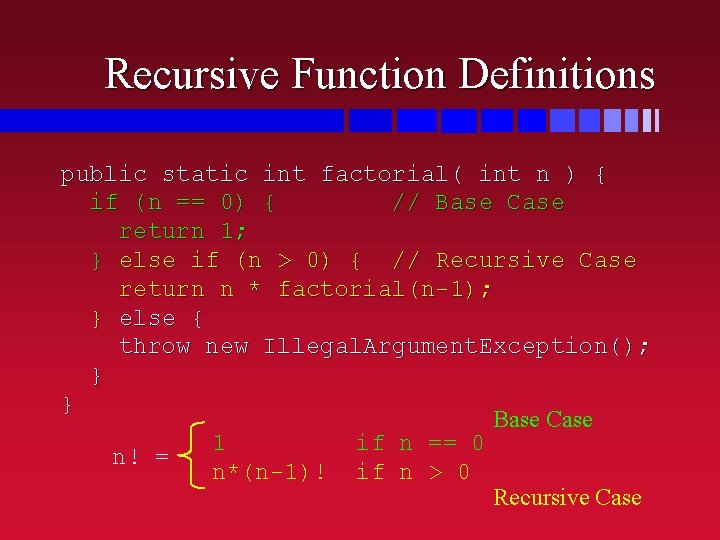Recursive Function Definitions public static int factorial( int n ) { if (n == 0) { // Base Case return 1; } else if (n > 0) { // Recursive Case return n * factorial(n-1); } else { throw new Illegal. Argument. Exception(); } } Base Case 1 if n == 0 n! = n*(n-1)! if n > 0 Recursive CaseCalling a Recursive Function n Just like calling any other function System. out. println( factorial(5) ); 120 n The function returns the factorial of what you give it s because that’s what it does s it returns the factorial every time you call it s including when you call it inside its definition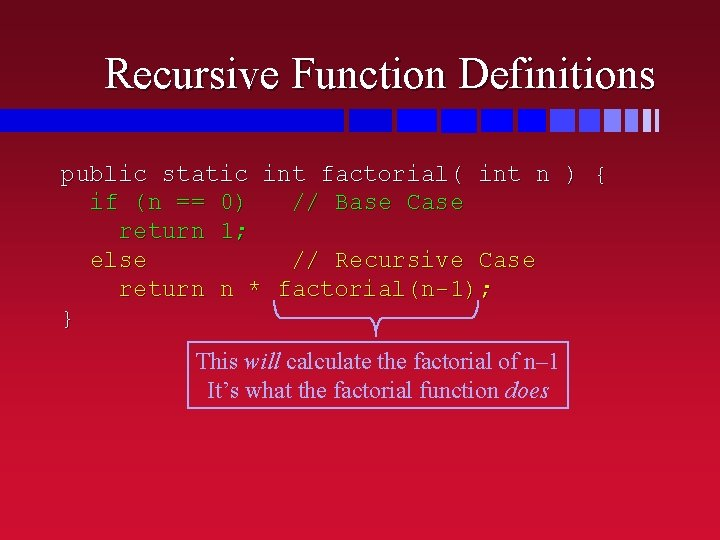Recursive Function Definitions public static int factorial( int n ) { if (n == 0) // Base Case return 1; else // Recursive Case return n * factorial(n-1); } This will calculate the factorial of n– 1 It’s what the factorial function does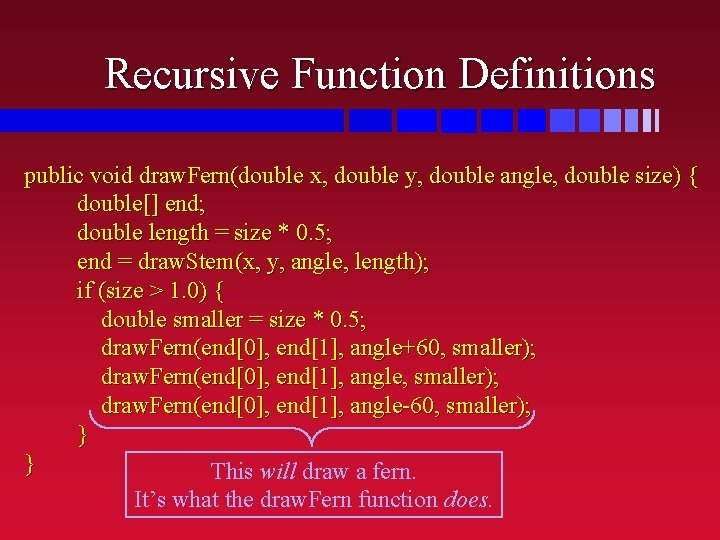Recursive Function Definitions public void draw. Fern(double x, double y, double angle, double size) { double[] end; double length = size * 0. 5; end = draw. Stem(x, y, angle, length); if (size > 1. 0) { double smaller = size * 0. 5; draw. Fern(end, end, angle+60, smaller); draw. Fern(end, end, angle-60, smaller); } } This will draw a fern. It’s what the draw. Fern function does.Exercise n Write a recursive function to calculate the (non-negative integer) power of an integer s Hint: it’ll look a LOT like the one for factorial nk = 1 n*nk-1 if k == 0 if k > 0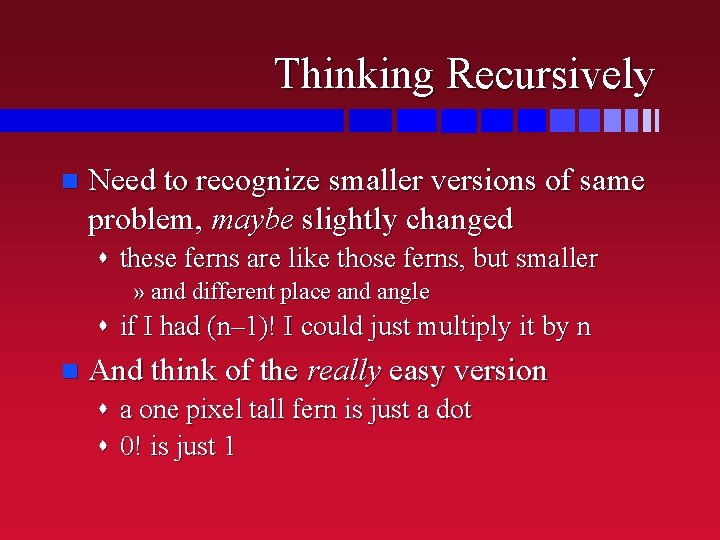Thinking Recursively n Need to recognize smaller versions of same problem, maybe slightly changed s these ferns are like those ferns, but smaller » and different place and angle s if I had (n– 1)! I could just multiply it by n n And think of the really easy version s a one pixel tall fern is just a dot s 0! is just 1Coding Recursively n Remember the two imperatives: s SMALLER! » when you call the method inside itself, one of the arguments has to be smaller than it was s STOP! » if the argument that gets smaller is very small (usually 0 or 1), then don’t do the recursion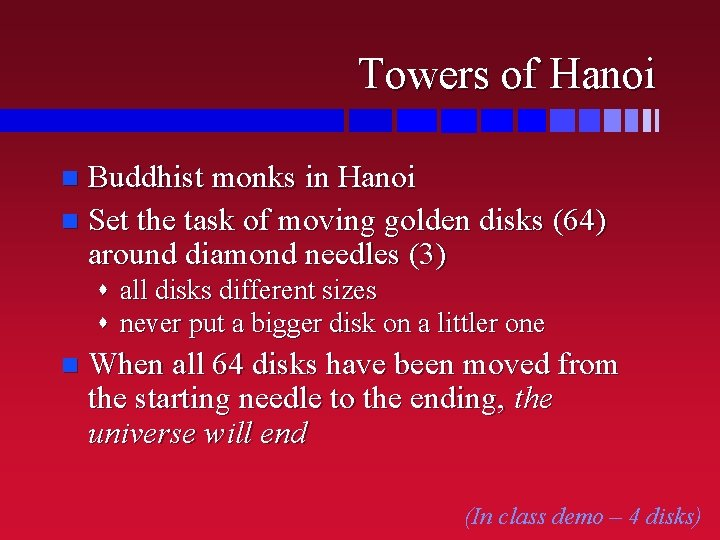Towers of Hanoi Buddhist monks in Hanoi n Set the task of moving golden disks (64) around diamond needles (3) n s all disks different sizes s never put a bigger disk on a littler one n When all 64 disks have been moved from the starting needle to the ending, the universe will end (In class demo – 4 disks)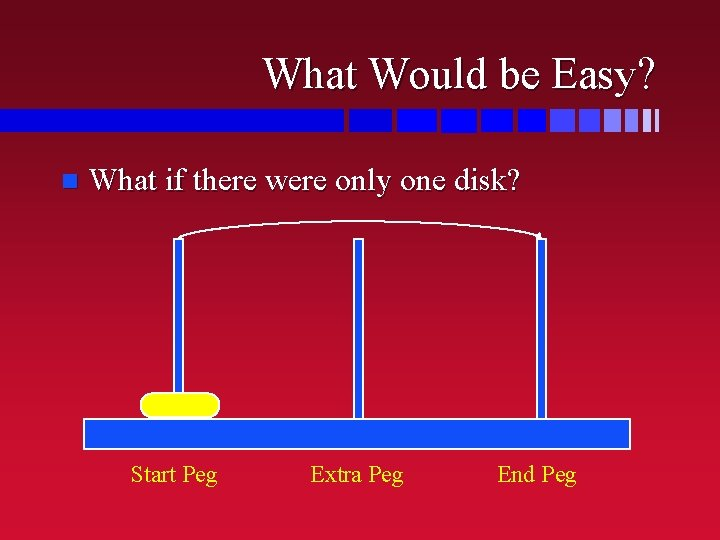What Would be Easy? n What if there were only one disk? Start Peg Extra Peg End PegA Little Less Easy n What if there were two disks? 1) Move one disk “out of the way” Start Peg 2) Move other disk to the end post Extra Peg 3) Move first disk to the end post End Peg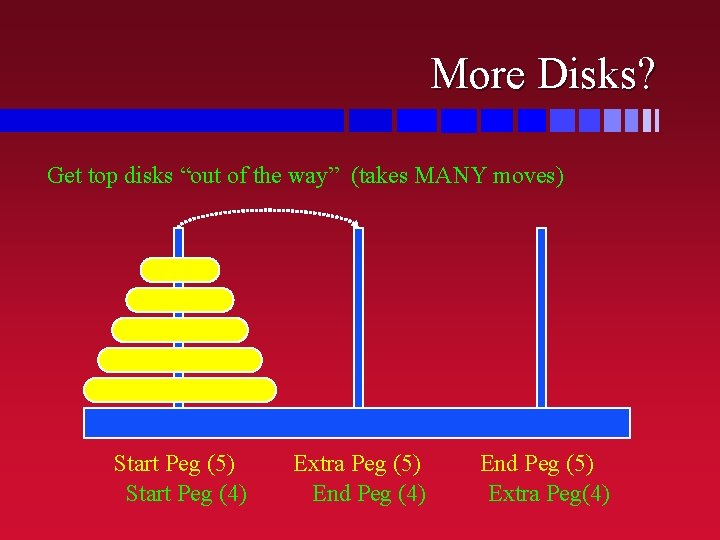More Disks? Get top disks “out of the way” (takes MANY moves) Start Peg (5) Start Peg (4) Extra Peg (5) End Peg (4) End Peg (5) Extra Peg(4)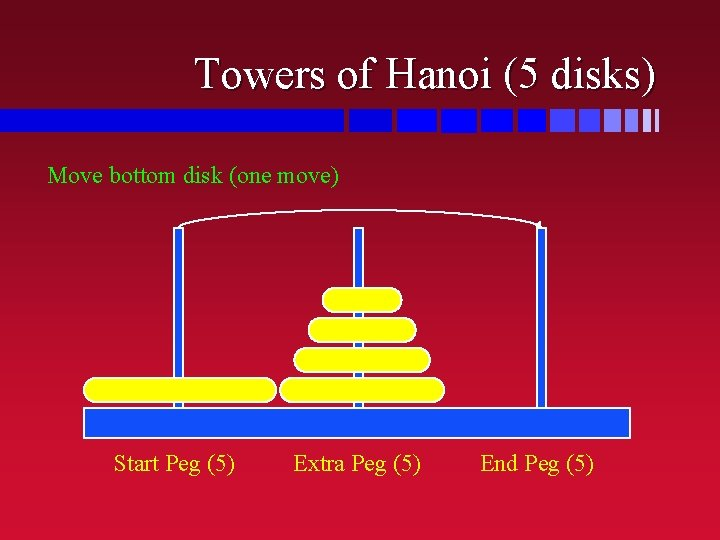Towers of Hanoi (5 disks) Move bottom disk (one move) Start Peg (5) Extra Peg (5) End Peg (5)Towers of Hanoi (5 disks) Finish moving the top disks over to the end peg (MANY moves) Start Peg (5) Extra Peg (4) Extra Peg (5) Start Peg (4) End Peg (5) End Peg(4)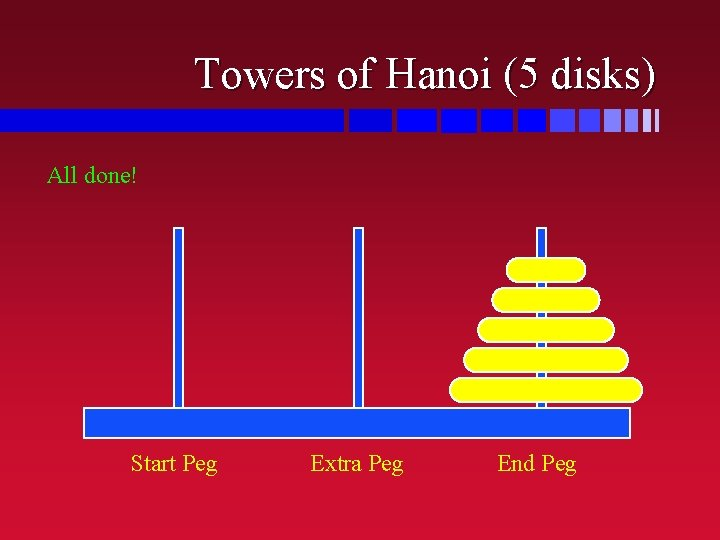Towers of Hanoi (5 disks) All done! Start Peg Extra Peg End PegTowers of Hanoi (Version 1) n If there’s only one disk s move it to the end peg n Else s move the top disks out of the way » extra peg becomes end peg for one less disks s move the bottom disk to the end peg s move the top disks onto the end peg » start peg becomes extra pegTowers of Hanoi (3 disks) Move 3 disks here Move 2 disks out of the way Move 1 disk out of the way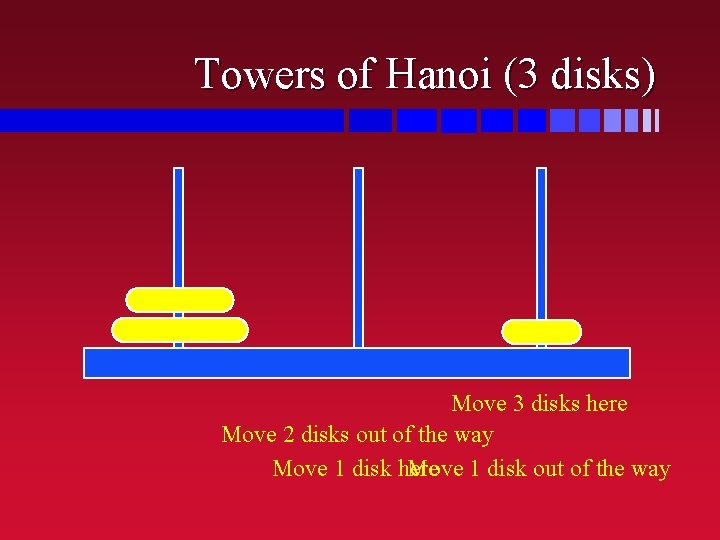Towers of Hanoi (3 disks) Move 3 disks here Move 2 disks out of the way Move 1 disk here Move 1 disk out of the way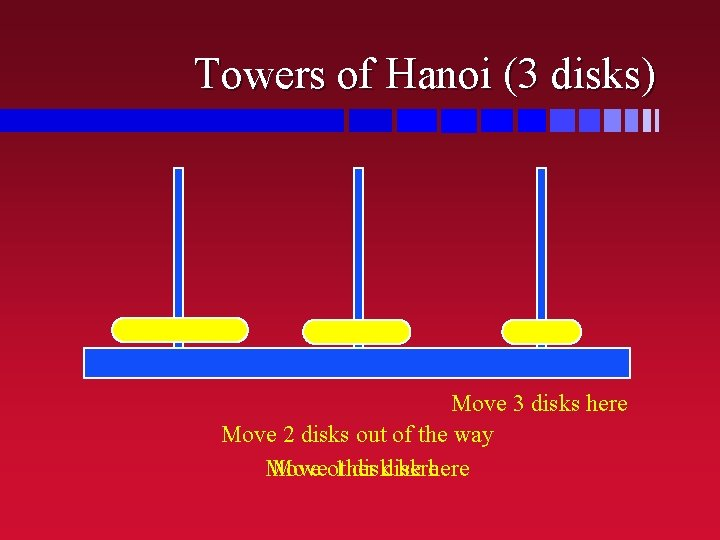Towers of Hanoi (3 disks) Move 3 disks here Move 2 disks out of the way Moveother 1 disk here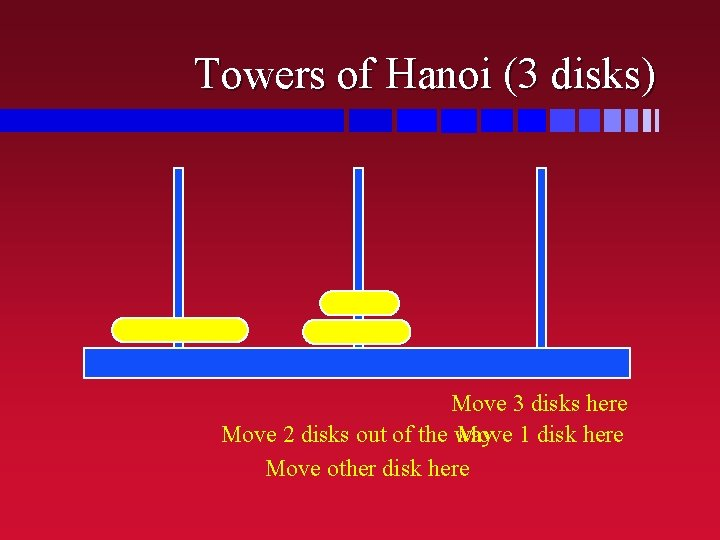Towers of Hanoi (3 disks) Move 3 disks here Move 2 disks out of the way Move 1 disk here Move other disk hereTowers of Hanoi (3 disks) Move 3 disks here Move 21 disks disk here Move 1 disk out of the way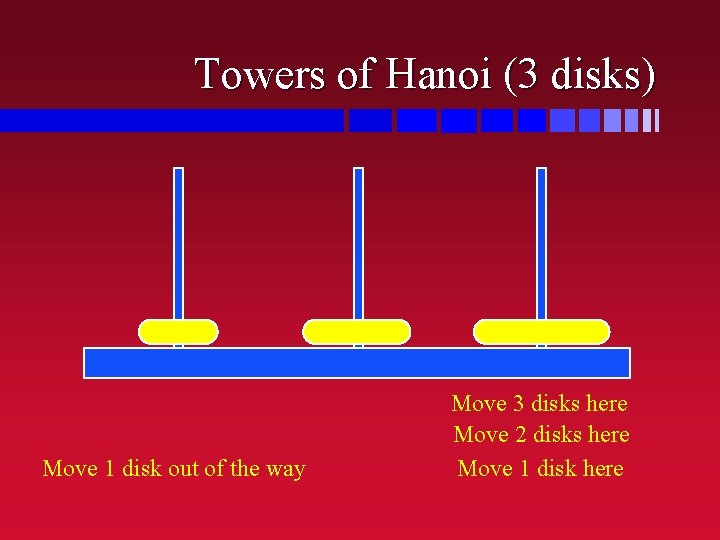Towers of Hanoi (3 disks) Move 1 disk out of the way Move 3 disks here Move 2 disks here Move 1 disk hereTowers of Hanoi (3 disks) Move 3 disks here Move 2 disks here Moveother 1 disk here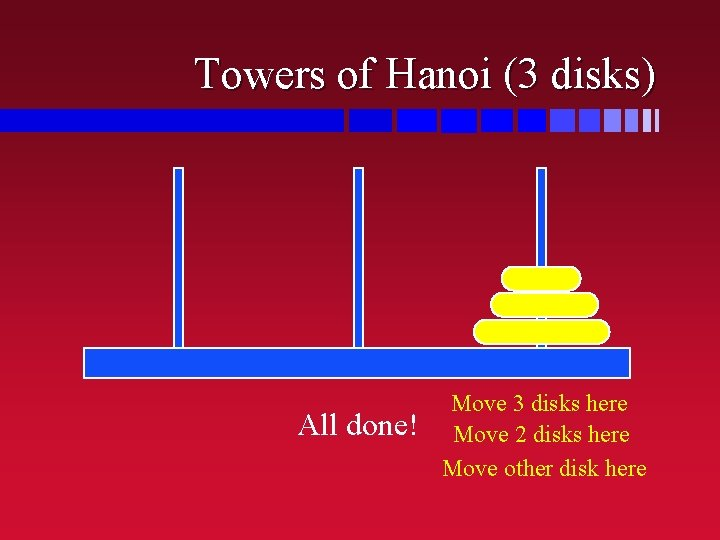Towers of Hanoi (3 disks) All done! Move 3 disks here Move 2 disks here Move other disk here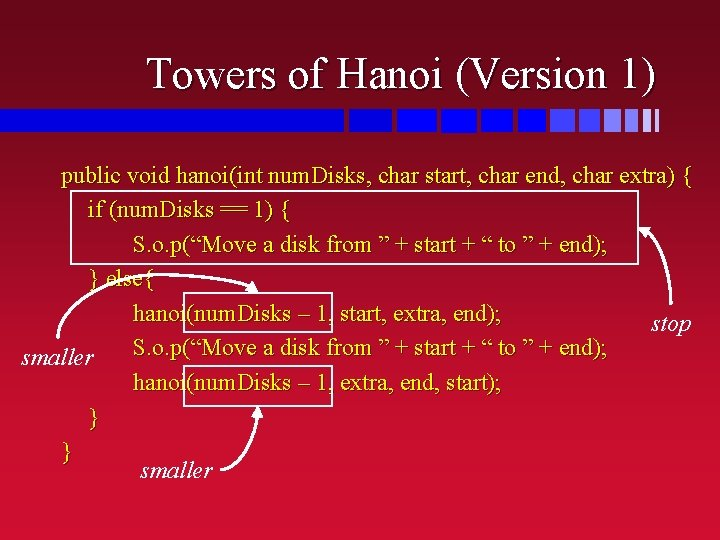Towers of Hanoi (Version 1) public void hanoi(int num. Disks, char start, char end, char extra) { if (num. Disks == 1) { S. o. p(“Move a disk from ” + start + “ to ” + end); } else{ hanoi(num. Disks – 1, start, extra, end); stop S. o. p(“Move a disk from ” + start + “ to ” + end); smaller hanoi(num. Disks – 1, extra, end, start); } } smaller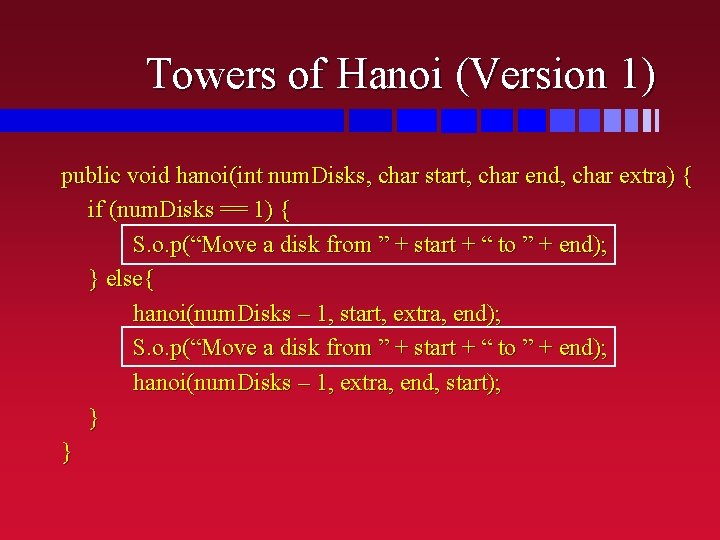Towers of Hanoi (Version 1) public void hanoi(int num. Disks, char start, char end, char extra) { if (num. Disks == 1) { S. o. p(“Move a disk from ” + start + “ to ” + end); } else{ hanoi(num. Disks – 1, start, extra, end); S. o. p(“Move a disk from ” + start + “ to ” + end); hanoi(num. Disks – 1, extra, end, start); } }What’s Easier than One Disk? n With no disks, there’d be nothing to do! s with one disk: » move zero disks out of the way » move bottom disk to end peg » mover zero disks onto bottom disk n Exercise s Towers of Hanoi (version 2) » simplify the code from the previous slide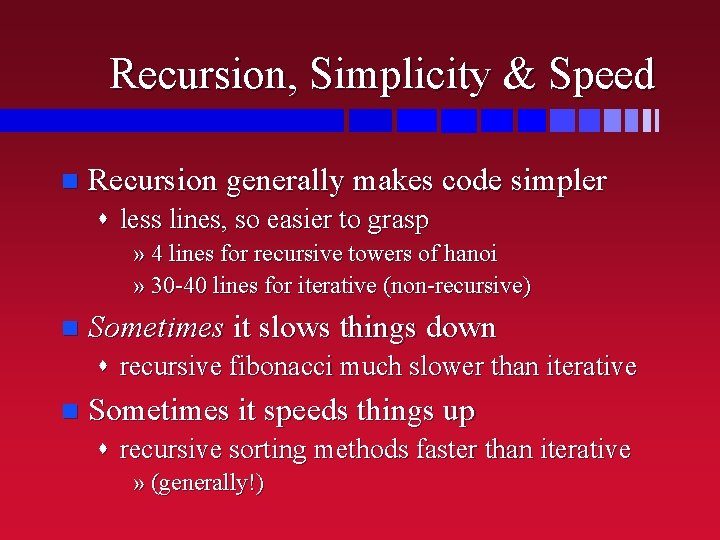Recursion, Simplicity & Speed n Recursion generally makes code simpler s less lines, so easier to grasp » 4 lines for recursive towers of hanoi » 30 -40 lines for iterative (non-recursive) n Sometimes it slows things down s recursive fibonacci much slower than iterative n Sometimes it speeds things up s recursive sorting methods faster than iterative » (generally!)Recursive Sorting Method n Merge sort a pile of student papers s if there’s more than one paper in this pile » divide it into two equal(ish) piles » merge-sort each smaller pile » merge the results back together n Much faster than inserting each paper into a sorted pile one at a time s especially on a computerBut Some Things are Just Slow Doesn’t matter how you do them, they take a long time n Towers of Hanoi n s if it takes 1 second to move each disk each time s how long until all 64 moved to the final peg? » 1 hour? » 1 day? » 1 year? » 100 years? » a million years? n disks 2 n – 1 steps 264 – 1 seconds… …about 585 billion years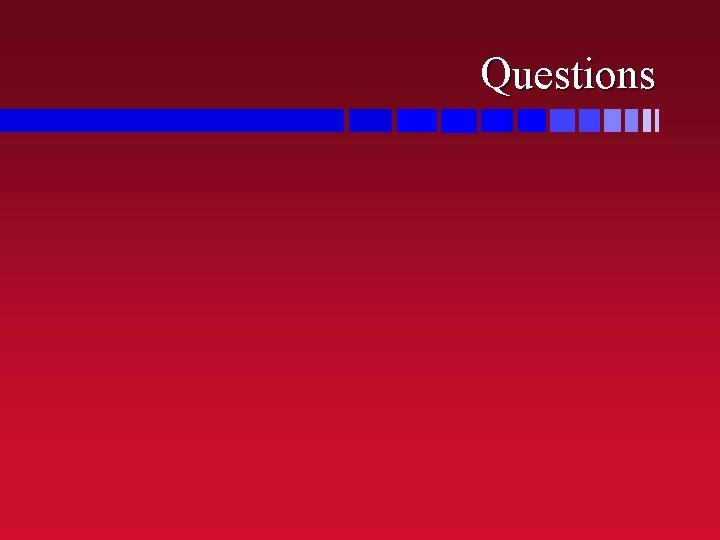Questions